# Difference between revisions of "Symmetric channel"

A communication channel whose transition function possesses some kind of symmetry. A homogeneous discrete time memoryless channel with finite alphabetsand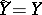of input and output letters, respectively, and defined by a matrix of transition probabilities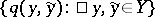is called a symmetric channel if: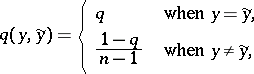(*)

whereis the number of elements of,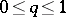. The most studied example of a memoryless symmetric channel is the binary symmetric channel with matrix of transition probabilities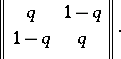For symmetric channels, many important information-theoretic characteristics can either be calculated explicitly or their calculation can be substantially simplified in comparison with non-symmetric channels. For example, for a memoryless symmetric channel with matrixof the form (*) the capacity(cf. Transmission rate of a channel) is given by the equationFor references see ,

cited under Communication channel.